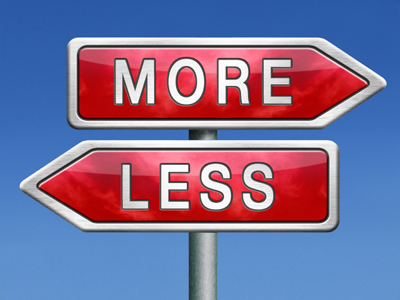If you are adding then you get more. If you are taking away then you get less.

# Year 1 Calculation - Addition and Subtraction Language

This quiz addresses the requirements of the National Curriculum KS1 Maths and Numeracy for children aged 5 and 6 in year 1. Specifically this quiz is aimed at the section dealing with developing the concept of addition and subtraction.

In Year 1 children are taught the language associated with the number operations of addition and subtraction, which is wide and varied. It can also be confusing - for example, to find the difference between two numbers, it's possible to count up from the lower to the higher number (effectively adding) or count down from the higher to the lower (subtracting)! It's worth knowing the key language associated with the concept of addition or subtraction. This will be especially useful when tackling word problems.

Question 1
What would you do to solve this:
Seven plus eight =
Take 7 away from 8
Take 8 away from 7
Subtract 7 from 8
Plus is another word for add
Question 2
Which words tell you this is an addition:
I had three balls and I bought six more. How many did I have altogether?
Three and six
More and altogether
Balls and many
Bought and have
More and altogether suggest adding up
Question 3
Which word could be placed in the space?
16 ____ 4 gives an answer of 12.
divided by
plus
minus
16 - 4 = 12. Minus is another word for subtract
Question 4
Which of the following could be used for addition or subtraction?
minus
the difference between
plus
subtract
The difference between two numbers can be found by either adding or subtracting
Question 5
Which of the following is to do with subtraction?
take away
totalling
in total
Taking one number away from another is subtracting
Question 6
Which of the following is to do with addition?
sharing
put together
take away
multiply
Putting two or more groups together is the same as adding them
Question 7
Which of the following is to do with subtraction?
less than
plus
altogether
The other words all mean to add
Question 8
Which words could be placed in the space?
8 ____ 11 gives an answer of 3.
multiplied by
divided by
subtracted from
Sometimes the number to be subtracted can come first in the number sentence
Question 9
Which of the following is to do with addition?
subtract
take
minus
total
A total is the sum of two or more groups
Question 10
Which word could be placed in the space?
12 ____ 8 gives an answer of 20.
subtract
plus
minus
multiplied by
Plus is another word for add